# Multiplicative Functions

Definition 3.1   A function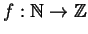is multiplicative if, whenever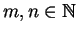and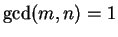, we have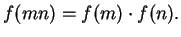Recall that the Euler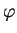-function is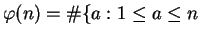and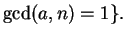Proposition 3.2is a multiplicative function.

Proof. Suppose thatand. Consider the map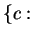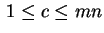and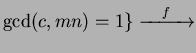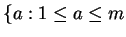and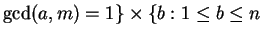and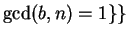defined by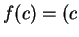mod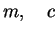mod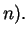The map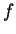is injective: If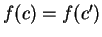, then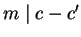and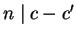, so, since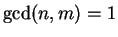,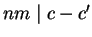, so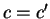.

The mapis surjective: Given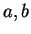with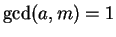,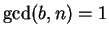, the Chinese Remainder Theorem implies that there exists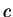with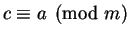and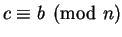. We may assume that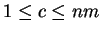, ans sinceand, we must have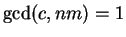. Thus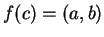.

Becauseis a bijection, the set on the left has the same size as the product set on the right. Thus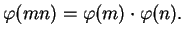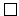Example 3.3   The proposition makes it easier to compute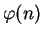. For example,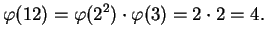Also, for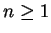, we have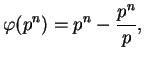since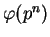is the number of numbers less than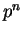minus the number of those that are divisible by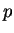. Thus, e.g.,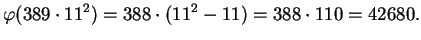Thefunction is also available in PARI:
  ? eulerphi(389*11^2)
%15 = 42680


Question 3.4   Is computing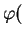1000 digit number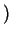really easy or really hard?